Select Page

# MCQ Answers for CBSE 12 Science Maths Integrals in English

MCQ Answers for CBSE 12 Science Maths Integrals in English to enable students to get Answers in a narrative video format for the specific question.

Expert Teacher provides MCQ Answers for CBSE 12 Science Maths Integrals through Video Answers in English language. This video solution will be useful for students to understand how to write an answer in exam in order to score more marks. This teacher uses a narrative style for a question from Integrals not only to explain the proper method of answering question, but deriving right answer too.

Please find the question below and view the Answer in a narrative video format.

Question:

## Similar Questions from CBSE, 12th Science, Maths, Integrals

Question 1 : Evaluate :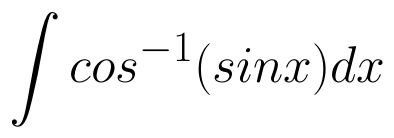. (View Answer Video)

Question 2 : Evaluate the following :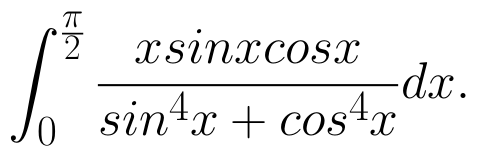(View Answer Video)

Question 3 : Evaluate :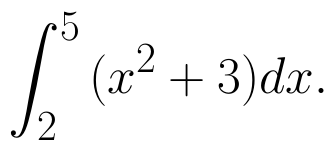(View Answer Video)

Question 4 : Evaluate :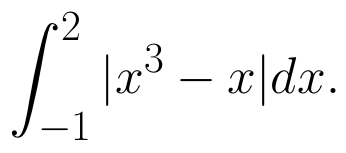(View Answer Video)

Question 5 : Find :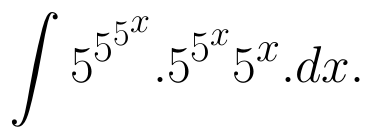(View Answer Video)

### Application of Integrals

Question 1 : Using the method of integration, find the area of the region bounded by the lines 3x - 2y + 1 = 0, 2x + 3y -21 = 0 and x - 5y + 9 = 0. (View Answer Video)

Question 2 : Find the area of the region enclosed by the parabola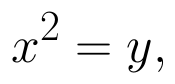the line y = x + 2. (View Answer Video)

Question 3 : Find the area of the smaller part of the circle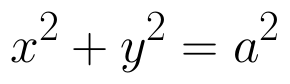cut off by the line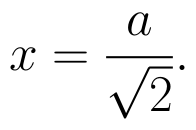(View Answer Video)

Question 4 : Using integration find the area of the triangular region whose sides have the equations y = 2x + 1, y = 3x + 1 and x = 4. (View Answer Video)

Question 5 : Find the area enclosed by the parabola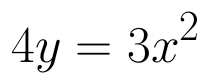and the line 2y = 3x + 12.   (View Answer Video)

### Application of Derivatives

Question 1 : The slope of the tangent to the curve: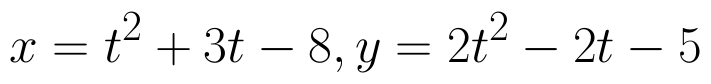Question 2 : The maximum value of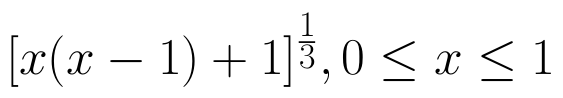is, (View Answer Video)

Question 3 : It is given that  at x=1, the function attains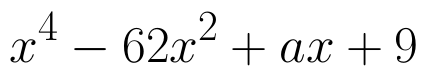its maximum value on the interval[0,2]. Find the value of a? (View Answer Video)

Question 4 : The line y = mx + 1 is a tangent to the curve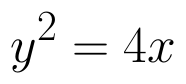if the value of m is: (View Answer Video)

Question 5 : The slope of the normal to the curve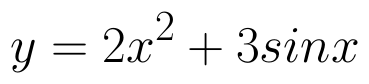at x = 0 is :
Question 1 : Functions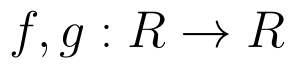are defined respectively, by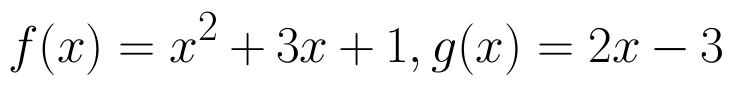, find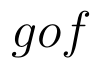(View Answer Video)
Question 4 : Functionsare defined respectively, by, find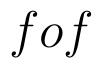(View Answer Video)